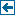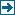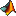Master index Index for Applications/SpinW/m_files# Index for iFit/Applications/SpinW/m_files

## Matlab files in this directory:gm_planar planar magnetic structure constraint functiongm_spherical3d magnetic structure constraint function with spherical parameterisationsw_addobject adds a graphical object to a selected figuresw_addsym saves user defined symmetry operatorssw_animate animates normal magnon modessw_annealfigure creates a figure for displaying the status of the annealing simulationsw_annealplot displays information about the annealing simulationsw_arrow draws a 3D arrowsw_atomdata returns information on elements stored in the atom.dat filesw_basismat determines allowed tensor components in a given point group symmetrysw_bose coefficient for boson correlation functions for different temperaturessw_cartesian creates a right handed Cartesian coordinate systemsw_circle creates an array of the 3D coordinates of the circle circumferencesw_circlesurf creates a circle surface in 3 dimensionssw_cmod modulo one with tolerancesw_colorname generates RGB code from color name stringsw_cone creates the surface of a cone in 3 dimensionsw_conv OUTDATED, use the new function sw_egrid instead.sw_converter converts energy and momentum units for a given particlesw_cylinder creates the surface of a cylinder in 3Dsw_draw plots additional object onto the crystal structuresw_drawpoly draws polyhedra around atoms on the structure plotsw_econtract converts (Q,omega) values to Qm values for diffraction instrumentsw_egrid creates energy for spectrum color plotsw_ellcylinder creates the surface of an elliptic cylindersw_ellipse creates an array of the 3D coordinates of the ellipse circumferencesw_extendlattice creates superlatticesw_filelist lists spinw data in the Matlab workspace or in a .mat filesw_freemem gives the amount of free RAM in bytessw_fstat calculates termodynamical averages during an annealing simulationsw_fsub simple graph vertex coloringsw_genatpos generates symmetry equivalent atomic positionssw_gencoord calculates all symmetry operators for a given space groupsw_gensym returns symmetry operators of a given space groupsw_gensymcoupling generates all equivalent couplings, using space group symmetrysw_getfighandle returns the handle of the active structure plot windowsw_getobject returns objects handles with given 'Tag' propertysw_handlelightsw_idata creates iData objectsw_initialize initializes sw library and cleans symmetry.dat file from user entriessw_instrument includes instrumental factors into the calculated spectrumsw_intsf integrates the structure factor along given Q directionssw_label returns axis labels for spectrum plotsw_magdomain calculates the spin-spin correlation function for magnetic domainssw_mattype determines the type of square input matrixsw_mff returns the magnetic form factor values and the coefficientssw_mirror mirrors a 3D vectorsw_model creates different predefined spin modelssw_multicolor creates RGB color data for multiple 2D overlapping plotssw_neutron calculates neutron scattering intensity for spin wave spectrumsw_nvect determines the best normal vector for the set of vectorssw_omegasum removes degenerate and ghost magnon modes from spectrumsw_orbital returns a polygon of selected hydrogen orbitalssw_parstr parses input stringsw_plotsf plots the structure factor in the selected Q range in 1D or 2Dsw_plotspec plots spin wave spectrumsw_pointsym determines point group symmetry of a space group at a given positionsw_qscan creates linear scans between Q points in 3Dsw_quadell calculates and plots the parameters of an ellipsoid from a quadratic formsw_readparam parse input arguments (option, value pairs)sw_readspec read spin wave dispersion data from filesw_res reads a tabulated energy resolution from a file and fits with polynomialsw_rootdir gives the path to the SpinW codesw_rot rotates vectors around arbitrary axis in 3Dsw_spinmotion calculates the amplitude and phase of spin motionsw_status timer function that displays also the remaining timesw_structfigure creates figure for crystal structure plotsw_symgetgen creates the generators from a list of symmetry operatorssw_symorder determine the order of the symmetry operatorsw_t2g plots t2g electron orbital surfacessw_uniquetol returns the unique column vectors within tolerancesw_update updates the SpinW installation from the internetsw_version returns the installed version of SpinW

## Subsequent directories:

Generated on Mon 26-Nov-2018 15:08:24 by m2html © 2005. iFit (c) E.Farhi/ILL EUPL 1.1